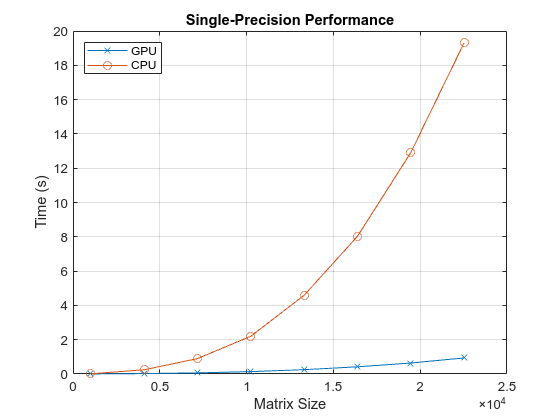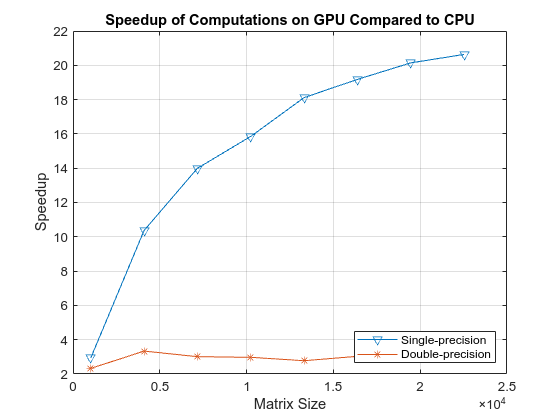# Benchmark A\b by Using GPU Coder

This example shows how to benchmark solving a linear system by generating GPU code. Use matrix left division, also known as `mldivide` or the backslash operator (\), to solve the system of linear equationsfor(that is, compute).

### Prerequisites

• CUDA® enabled NVIDIA® GPU with compute capability 3.5 or higher.

• NVIDIA CUDA toolkit and driver.

• Environment variables for the compilers and libraries. For information on the supported versions of the compilers and libraries, see Third-party Products. For setting up the environment variables, see Setting Up the Prerequisite Products.

### Verify GPU Environment

To verify that the compilers and libraries necessary for running this example are set up correctly, use the `coder.checkGpuInstall` function.

```envCfg = coder.gpuEnvConfig('host'); envCfg.BasicCodegen = 1; envCfg.Quiet = 1; coder.checkGpuInstall(envCfg); ```

### Determine the Maximum Data Size

0Choose the appropriate matrix size for the computations by specifying the amount of system memory in GB available to the CPU and the GPU. The default value is based only on the amount of memory available on the GPU. You can specify a value that is appropriate for your system.

```g = gpuDevice; maxMemory = 0.25*g.AvailableMemory/1024^3; ```

### The Benchmarking Function

This example benchmarks matrix left division (\) including the cost of transferring data between the CPU and GPU, to get a clear view of the total application time when using GPU Coder™. The application time profiling must not include the time to create sample input data. The `genData.m` function separates generation of test data from the entry-point function that solves the linear system.

```type getData.m ```
```function [A, b] = getData(n, clz) % Copyright 2017-2019 The MathWorks, Inc. fprintf('Creating a matrix of size %d-by-%d.\n', n, n); A = rand(n, n, clz) + 100*eye(n, n, clz); b = rand(n, 1, clz); end ```

### The Backslash Entry-Point Function

The `backslash.m` entry-point function encapsulates the (\) operation for which you want to generate code.

```type backslash.m ```
```function [x] = backslash(A,b) %#codegen % Copyright 2017-2019 The MathWorks, Inc. coder.gpu.kernelfun(); x = A\b; end ```

### Generate the GPU Code

Create a function to generate the GPU MEX function based on the particular input data size.

```type genGpuCode.m ```
```function [] = genGpuCode(A, b) % Copyright 2017-2019 The MathWorks, Inc. cfg = coder.gpuConfig('mex'); evalc('codegen -config cfg -args {A,b} backslash'); end ```

### Choose a Problem Size

The performance of the parallel algorithms that solve a linear system depends greatly on the matrix size. This example compares the performance of the algorithm for different matrix sizes.

```% Declare the matrix sizes to be a multiple of 1024. sizeLimit = inf; if ispc sizeLimit = double(intmax('int32')); end maxSizeSingle = min(floor(sqrt(maxMemory*1024^3/4)),floor(sqrt(sizeLimit/4))); maxSizeDouble = min(floor(sqrt(maxMemory*1024^3/8)),floor(sqrt(sizeLimit/8))); step = 1024; if maxSizeDouble/step >= 10 step = step*floor(maxSizeDouble/(5*step)); end sizeSingle = 1024:step:maxSizeSingle; sizeDouble = 1024:step:maxSizeDouble; numReps = 5; ```

### Compare Performance: Speedup

Use the total elapsed time as a measure of performance because that enables you to compare the performance of the algorithm for different matrix sizes. Given a matrix size, the benchmarking function creates the matrix `A` and the right-side `b` once, and then solves `A\b` a few times to get an accurate measure of the time it takes.

```type benchFcnMat.m ```
```function time = benchFcnMat(A, b, reps) % Copyright 2017-2019 The MathWorks, Inc. time = inf; % Solve the linear system a few times and take the best run for itr = 1:reps tic; matX = backslash(A, b); tcurr = toc; time = min(tcurr, time); end end ```

Create a different function for GPU code execution that invokes the generated GPU MEX function.

```type benchFcnGpu.m ```
```function time = benchFcnGpu(A, b, reps) % Copyright 2017-2019 The MathWorks, Inc. time = inf; gpuX = backslash_mex(A, b); for itr = 1:reps tic; gpuX = backslash_mex(A, b); tcurr = toc; time = min(tcurr, time); end end ```

### Execute the Benchmarks

When you execute the benchmarks, the computations can take a long time to complete. Print some intermediate status information as you complete the benchmarking for each matrix size. Encapsulate the loop over all the matrix sizes in a function to benchmark single- and double-precision computations.

Actual execution times can vary across different hardware configurations. This benchmarking was done by using MATLAB R2020a on a machine with a 6 core, 3.5GHz Intel® Xeon® CPU and an NVIDIA TITAN Xp GPU.

```type executeBenchmarks.m ```
```function [timeCPU, timeGPU] = executeBenchmarks(clz, sizes, reps) % Copyright 2017-2019 The MathWorks, Inc. fprintf(['Starting benchmarks with %d different %s-precision ' ... 'matrices of sizes\nranging from %d-by-%d to %d-by-%d.\n'], ... length(sizes), clz, sizes(1), sizes(1), sizes(end), ... sizes(end)); timeGPU = zeros(size(sizes)); timeCPU = zeros(size(sizes)); for i = 1:length(sizes) n = sizes(i); fprintf('Size : %d\n', n); [A, b] = getData(n, clz); genGpuCode(A, b); timeCPU(i) = benchFcnMat(A, b, reps); fprintf('Time on CPU: %f sec\n', timeCPU(i)); timeGPU(i) = benchFcnGpu(A, b, reps); fprintf('Time on GPU: %f sec\n', timeGPU(i)); fprintf('\n'); end end ```

Execute the benchmarks in single and double precision.

```[cpu, gpu] = executeBenchmarks('single', sizeSingle, numReps); results.sizeSingle = sizeSingle; results.timeSingleCPU = cpu; results.timeSingleGPU = gpu; [cpu, gpu] = executeBenchmarks('double', sizeDouble, numReps); results.sizeDouble = sizeDouble; results.timeDoubleCPU = cpu; results.timeDoubleGPU = gpu; ```
```Starting benchmarks with 9 different single-precision matrices of sizes ranging from 1024-by-1024 to 25600-by-25600. Size : 1024 Creating a matrix of size 1024-by-1024. Time on CPU: 0.011606 sec Time on GPU: 0.012707 sec Size : 4096 Creating a matrix of size 4096-by-4096. Time on CPU: 0.251755 sec Time on GPU: 0.061536 sec Size : 7168 Creating a matrix of size 7168-by-7168. Time on CPU: 0.879068 sec Time on GPU: 0.137024 sec Size : 10240 Creating a matrix of size 10240-by-10240. Time on CPU: 2.161492 sec Time on GPU: 0.274395 sec Size : 13312 Creating a matrix of size 13312-by-13312. Time on CPU: 4.235362 sec Time on GPU: 0.480827 sec Size : 16384 Creating a matrix of size 16384-by-16384. Time on CPU: 8.280482 sec Time on GPU: 0.761206 sec Size : 19456 Creating a matrix of size 19456-by-19456. Time on CPU: 11.975437 sec Time on GPU: 1.158090 sec Size : 22528 Creating a matrix of size 22528-by-22528. Time on CPU: 18.745547 sec Time on GPU: 1.673389 sec Size : 25600 Creating a matrix of size 25600-by-25600. Time on CPU: 27.993778 sec Time on GPU: 2.309414 sec Starting benchmarks with 7 different double-precision matrices of sizes ranging from 1024-by-1024 to 19456-by-19456. Size : 1024 Creating a matrix of size 1024-by-1024. Time on CPU: 0.026676 sec Time on GPU: 0.020420 sec Size : 4096 Creating a matrix of size 4096-by-4096. Time on CPU: 0.438594 sec Time on GPU: 0.175739 sec Size : 7168 Creating a matrix of size 7168-by-7168. Time on CPU: 1.653028 sec Time on GPU: 0.770956 sec Size : 10240 Creating a matrix of size 10240-by-10240. Time on CPU: 4.596732 sec Time on GPU: 2.075814 sec Size : 13312 Creating a matrix of size 13312-by-13312. Time on CPU: 8.706927 sec Time on GPU: 4.432075 sec Size : 16384 Creating a matrix of size 16384-by-16384. Time on CPU: 20.101956 sec Time on GPU: 8.063678 sec Size : 19456 Creating a matrix of size 19456-by-19456. Time on CPU: 27.399002 sec Time on GPU: 13.432378 sec ```

### Plot the Performance

Plot the results and compare the performance on the CPU and the GPU for single and double precision.

First, look at the performance of the backslash operator in single precision.

```fig = figure; ax = axes('parent', fig); plot(ax, results.sizeSingle, results.timeSingleGPU, '-x', ... results.sizeSingle, results.timeSingleCPU, '-o') grid on; legend('GPU', 'CPU', 'Location', 'NorthWest'); title(ax, 'Single-Precision Performance') ylabel(ax, 'Time (s)'); xlabel(ax, 'Matrix Size'); drawnow; ```Now, look at the performance of the backslash operator in double precision.

```fig = figure; ax = axes('parent', fig); plot(ax, results.sizeDouble, results.timeDoubleGPU, '-x', ... results.sizeDouble, results.timeDoubleCPU, '-o') legend('GPU', 'CPU', 'Location', 'NorthWest'); grid on; title(ax, 'Double-Precision Performance') ylabel(ax, 'Time (s)'); xlabel(ax, 'Matrix Size'); drawnow; ```Finally, look at the speedup of the backslash operator when comparing the GPU to the CPU.

```speedupDouble = results.timeDoubleCPU./results.timeDoubleGPU; speedupSingle = results.timeSingleCPU./results.timeSingleGPU; fig = figure; ax = axes('parent', fig); plot(ax, results.sizeSingle, speedupSingle, '-v', ... results.sizeDouble, speedupDouble, '-*') grid on; legend('Single-precision', 'Double-precision', 'Location', 'SouthEast'); title(ax, 'Speedup of Computations on GPU Compared to CPU'); ylabel(ax, 'Speedup'); xlabel(ax, 'Matrix Size'); drawnow; ```